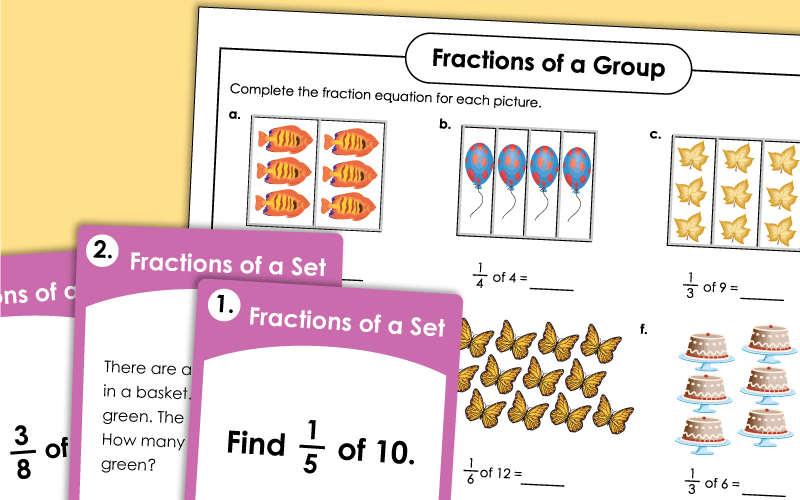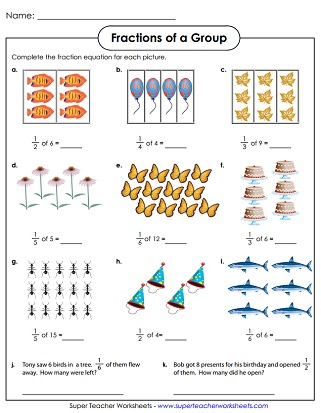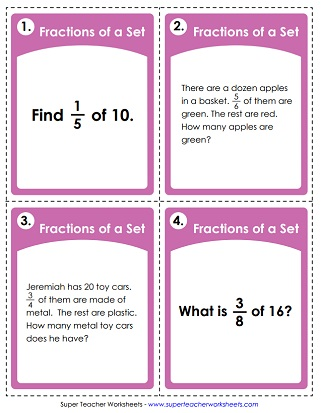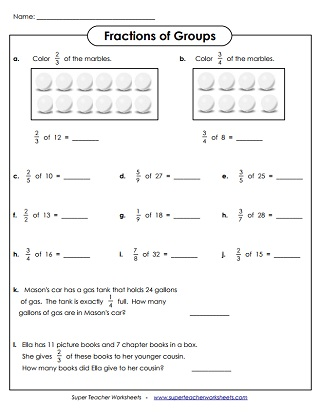# Fractional Parts of a Sets

This page has worksheets for teaching students about fractional parts of sets. (For example: What is one-third of 27?)## Fractions of SetsUnit Fractions Only(Basic Level)

Find the fractional part of each group; Includes only unit fractions; Easier version  (example: 1/5 of 15).
Use the illustrations to help find the fraction of each number; Unit fractions only.  (example: 1/3 of 9)
Color fractional part of each group of marbles; Includes unit fractions only; Easier version  (example: 1/3 of 21).

## Fractions of SetsAll Fractions(Advanced Level)

Find the fractional part of each group; Harder version  (example: 3/7 of 28).
Color fractions of each group of marbles; Harder version  (example: 4/7 of 21).
This file has 30 task cards with problems that require students to calculate fraction of a set. (example: 3/4 of 24).
More Fraction Worksheets

This section of the site covers equivalent fractions, simplifying fractions, mixed numbers, reciprocal fractions, adding and subtracting fractions, as well as multiplying and dividing fractions.

## Sample Printable ImagesMy Account
Site Information